Question

# Gaseous ethane (CH3CH3) will react with gaseous oxygen (O2) to produce gaseous carbon dioxide (CO2) and...

Gaseous ethane (CH3CH3) will react with gaseous oxygen (O2) to produce gaseous carbon dioxide (CO2) and gaseous water (H2O). Suppose 23. g of ethane is mixed with 124. g of oxygen. Calculate the maximum mass of carbon dioxide that could be produced by the chemical reaction. Round your answer to 2 significant digits.

From the balanced equation we can calculate the limiting reactant and the mol of CO2 formed.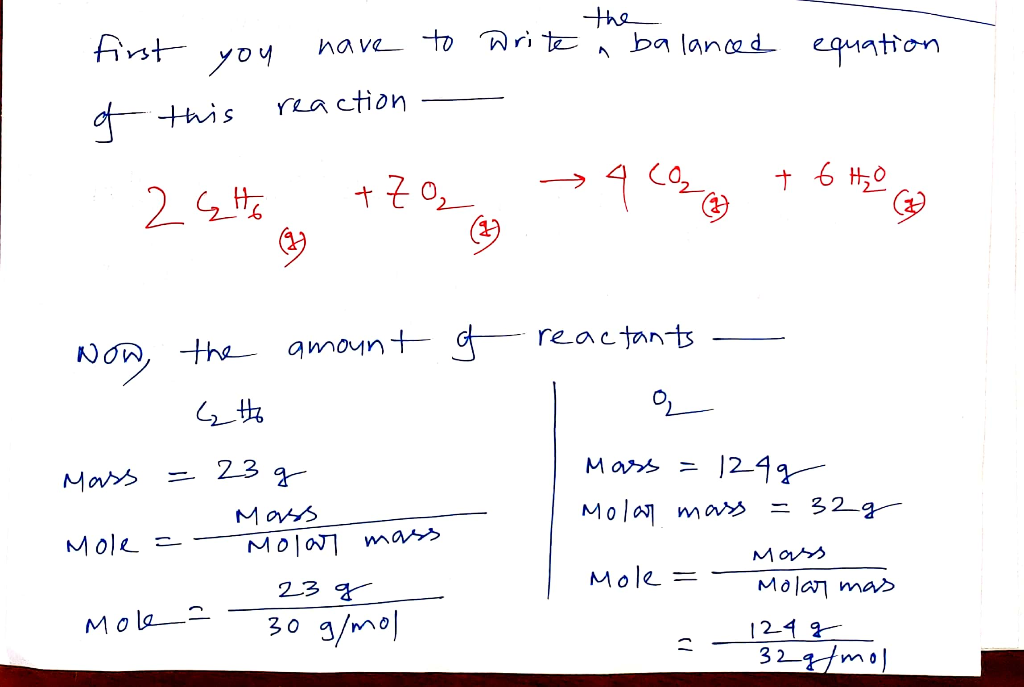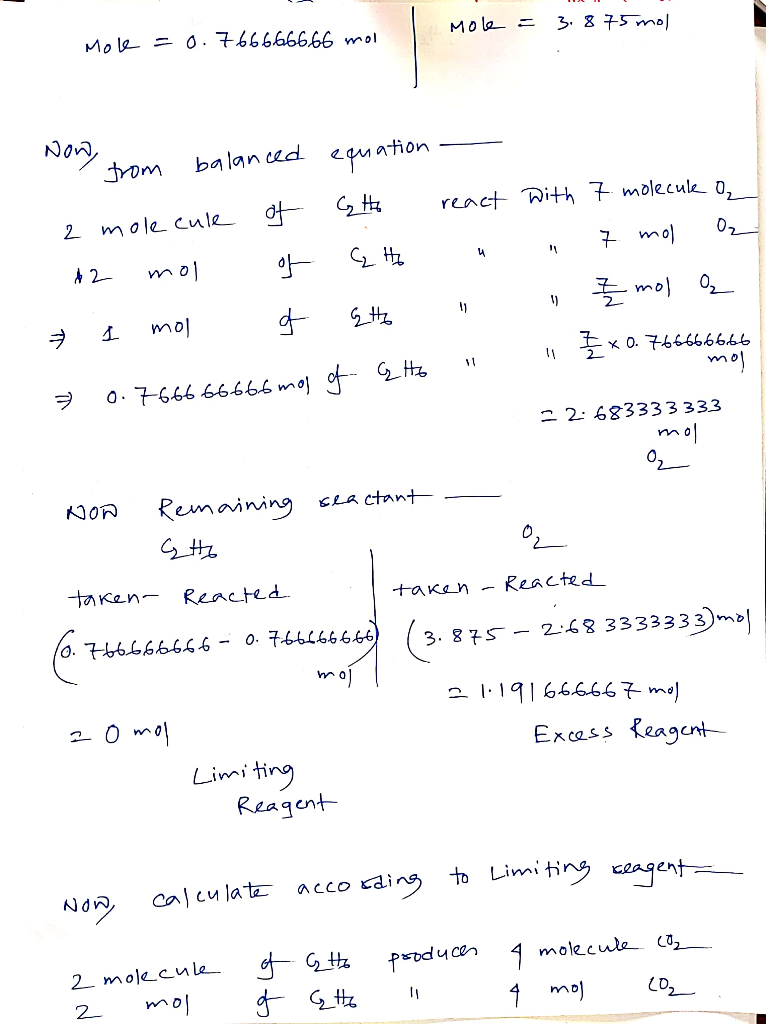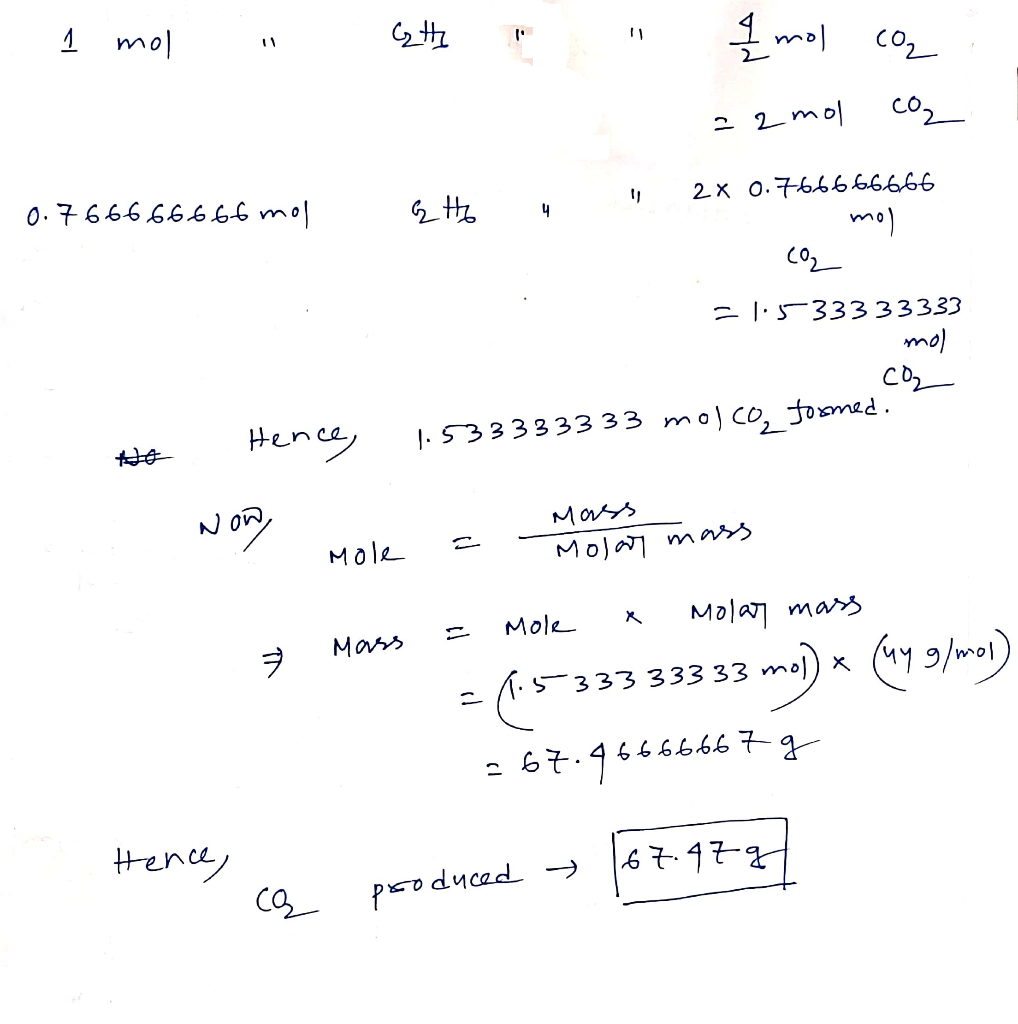#### Earn Coins

Coins can be redeemed for fabulous gifts.

Similar Homework Help Questions
• ### Gaseous ethane CH3CH3 will react with gaseous oxygen O2 to produce gaseous carbon dioxide CO2 and...

Gaseous ethane CH3CH3 will react with gaseous oxygen O2 to produce gaseous carbon dioxide CO2 and gaseous water H2O. Suppose 15. g of ethane is mixed with 105. g of oxygen. Calculate the maximum mass of water that could be produced by the chemical reaction. Round your answer to 2 significant digits.

• ### Gaseous ethane CH3CH3 will react with gaseous oxygen O2 to produce gaseous carbon dioxide CO2 and...

Gaseous ethane CH3CH3 will react with gaseous oxygen O2 to produce gaseous carbon dioxide CO2 and gaseous water H2O. Suppose 1.2 g of ethane is mixed with 3.27 g of oxygen. Calculate the maximum mass of water that could be produced by the chemical reaction. Be sure your answer has the correct number of significant digits.

• ### Gaseous ethane CH3CH3 will react with gaseous oxygen O2 to produce gaseous carbon dioxide CO2 and...

Gaseous ethane CH3CH3 will react with gaseous oxygen O2 to produce gaseous carbon dioxide CO2 and gaseous water H2O. Suppose 1.8 g of ethane is mixed with 12.6 g of oxygen. Calculate the maximum mass of water that could be produced by the chemical reaction. Be sure your answer has the correct number of significant digits.

• ### Gaseous ethane (CH3CH3) will react with gaseous oxygen (O2) to produce gaseous carbon dioxide (Co2) and...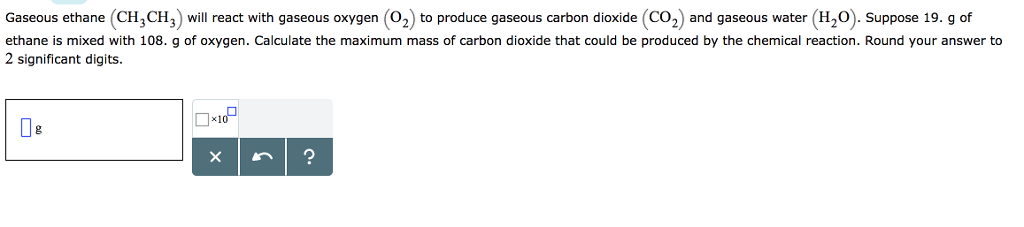Gaseous ethane (CH3CH3) will react with gaseous oxygen (O2) to produce gaseous carbon dioxide (Co2) and gaseous water (H20). Suppose 19. g of ethane is mixed with 108. g of oxygen. Calculate the maximum mass of carbon dioxide that could be produced by the chemical reaction. Round your answer to 2 significant digits ×1 De

• ### Gaseous ethane CH3CH3 will react with gaseous oxygen O2 to produce gaseous carbon dioxide CO2 and...

Gaseous ethane CH3CH3 will react with gaseous oxygen O2 to produce gaseous carbon dioxide CO2 and gaseous water H2O . Suppose 12.6 g of ethane is mixed with 35. g of oxygen. Calculate the minimum mass of ethane that could be left over by the chemical reaction. Round your answer to 2 significant digits.

• ### Gaseous ethane CH3CH3 will react with gaseous oxygen O2 to produce gaseous carbon dioxide CO2 and...

Gaseous ethane CH3CH3 will react with gaseous oxygen O2 to produce gaseous carbon dioxide CO2 and gaseous water H2O . Suppose 2.71 g of ethane is mixed with 19. g of oxygen. Calculate the minimum mass of ethane that could be left over by the chemical reaction. Be sure your answer has the correct number of significant digits.

• ### Gaseous ethane (CH,CH) will react with gaseous oxygen (O2) to produce gaseous carbon dioxide (CO2) and...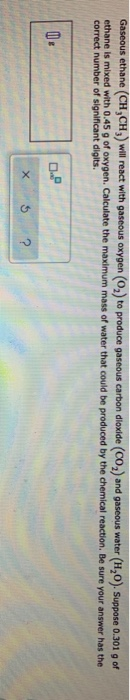Gaseous ethane (CH,CH) will react with gaseous oxygen (O2) to produce gaseous carbon dioxide (CO2) and gaseous water (HO). Suppose 0.301 g of ethane is mixed with 0.45 g of oxygen. Calculate the maximum mass of water that could be produced by the chemical reaction. Be sure your answer has the correct number of significant digits. 00

• ### 3. Gaseous CH4 will react with gaseous oxygen (O2) to produce gaseous carbon dioxide (CO2) and...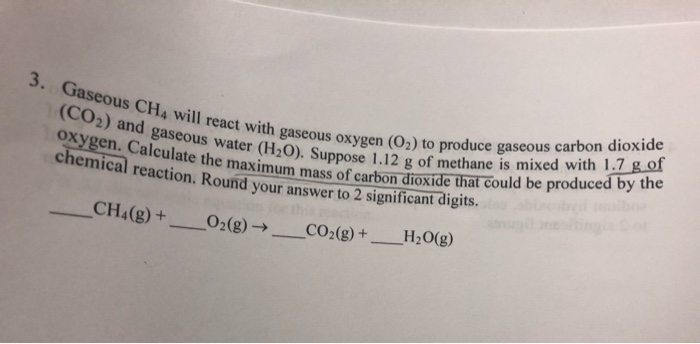3. Gaseous CH4 will react with gaseous oxygen (O2) to produce gaseous carbon dioxide (CO2) and gaseous water (H20). Suppose 1.12 g of methane is mixed with 1.7 g of Oxygen. Calculate the maximum mass of carbon dioxide that could be produced by the chemical reaction. Round your answer to 2 significant digits. CH4(g)+ 02 (g) ->_CO2(g) + H2O(g) 5. The following chemical reaction takes place in aqueous solutions: 2AGF(aq) + (NH4)2 CO 3 (aq) Ag, CO3 (aq) + 2NH4F(aq)...

• ### Gaseous methane CH4 will react with gaseous oxygen O2 to produce gaseous carbon dioxide CO2 and...

Gaseous methane CH4 will react with gaseous oxygen O2 to produce gaseous carbon dioxide CO2 and gaseous water H2O . Suppose 6.42 g of methane is mixed with 7.4 g of oxygen. Calculate the maximum mass of carbon dioxide that could be produced by the chemical reaction. Be sure your answer has the correct number of significant digits.

• ### Gaseous butane CH3CH22CH3 will react with gaseous oxygen O2 to produce gaseous carbon dioxide CO2 and...

Gaseous butane CH3CH22CH3 will react with gaseous oxygen O2 to produce gaseous carbon dioxide CO2 and gaseous water H2O. Suppose 26.2 g of butane is mixed with 36. g of oxygen. Calculate the maximum mass of carbon dioxide that could be produced by the chemical reaction. Be sure your answer has the correct number of significant digits.